## RD Sharma Class 9 Solutions Chapter 17 Constructions Ex 17.1

These Solutions are part of RD Sharma Class 9 Solutions. Here we have given RD Sharma Class 9 Solutions Chapter 17 Constructions Ex 17.1

Other Exercises

Question 1.
Find the area of a triangle whose sides are respectively 150 cm, 120 cm and 200 cm.
Solution:
Sides of triangle are 120 cm, 150 cm, 200 cm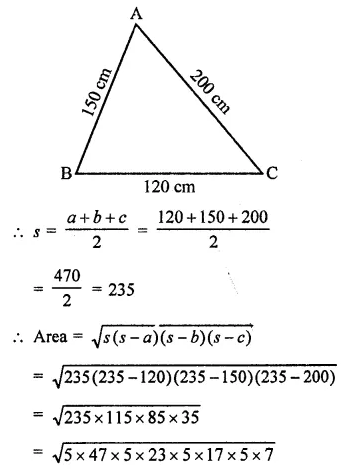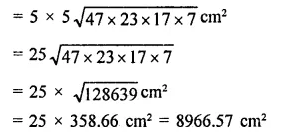Question 2.
Find the area of a triangle whose sides are 9 cm, 12 cm and 15 cm.
Solution:
Sides of a triangle are 9 cpi, 12 cm, 15 cmQuestion 3.
Find the area of a triangle two sides of which are 18 cm and 10 cm and the perimeter is 42 cm.
Solution:
Perimeter of a triangle = 42 cm
Two sides are 18 cm and 10 cm
Third side = 42 – (18 + 10)
= 42 – 28 = 14 cm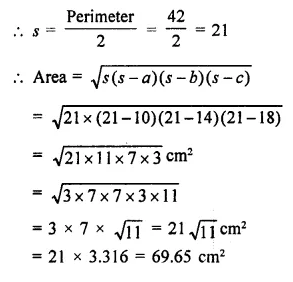Question 4.
In a ∆ABC, AB = 15 cm, BC = 13 cm and AC = 14 cm. Find the area of ∆ABC and hence its altitude on AC.
Solution:
Sides of triangle ABC are AB = 15 cm, BC = 13 cm, AC = 14 cm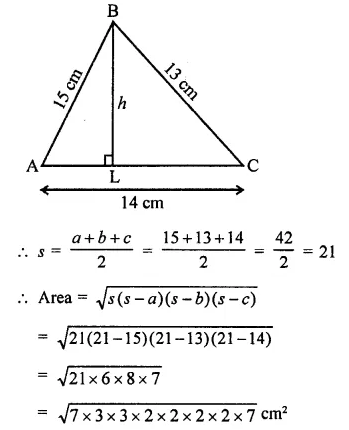Question 5.
The perimeter of a triangular field is 540 m and its sides are in the ratio 25 : 17 : 12. Find the area of the triangle. [NCERT]
Solution:
Perimeter of a triangle = 540 m
Ratio in sides = 25 : 17 : 12
Sum of ratios = 25 + 17 + 12 = 54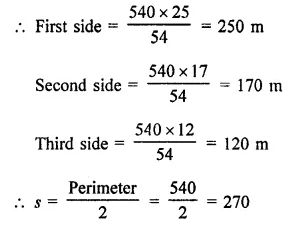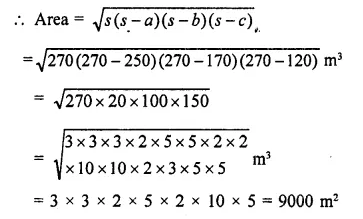Question 6.
The perimeter of a triangle is 300 m. If its sides are in the ratio 3:5:7. Find the area of the triangle. [NCERT]
Solution:
Perimeter of a triangle = 300 m
Ratio in the sides = 3 : 5 : 7
∴ Sum of ratios = 3 + 5 + 7= 15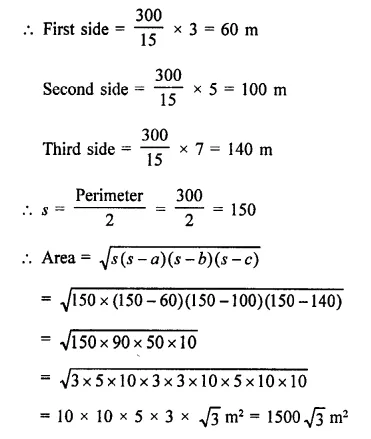Question 7.
The perimeter of a triangular field is 240 dm. If two of its sides are 78 dm and 50 dm, find the length of the perpendicular on the side of length 50 dm from the opposite vertex.
Solution:
Perimeter of a triangular field = 240 dm
Two sides are 78 dm and 50 dm
∴ Third side = 240 – (78 + 50)
= 240 – 128 = 112 dmQuestion 8.
A triangle has sides 35 cm, 54 cm and 61 cm long. Find its area. Also, find the smallest of its altitudes.
Solution:
Sides of a triangle are 35 cm, 54 cm, 61 cmQuestion 9.
The lengths of the sides of a triangle are in the ratio 3:4:5 and its perimeter is 144 cm. Find the area of the triangle and the height corresponding to the longest side.
Solution:
Ratio in the sides of a triangle = 3:4:5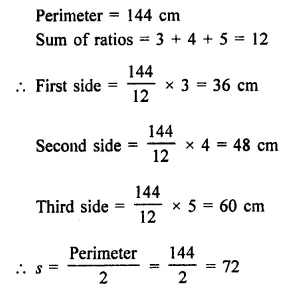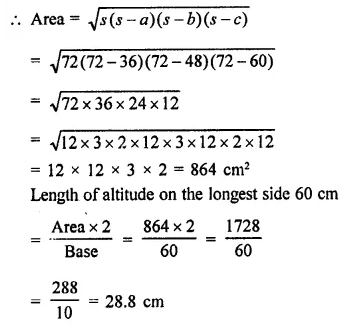Question 10.
The perimeter of an isosceles triangle is 42 cm and its base is (3/2) times each of the equal sides. Find the length of each side of the triangle, area of the triangle and the height of the triangle.
Solution:
Perimeter of an isosceles triangle = 42 cm
Base = $$\frac { 3 }{ 2 }$$ of its one of equal sides
Let each equal side = x, then 3
Base = $$\frac { 3 }{ 2 }$$ x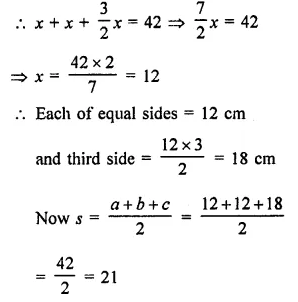Question 11.
Find the area of the shaded region in figure.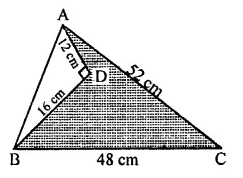Solution:
In ∆ABC, AC = 52 cm, BC = 48 cm
and in right ∆ADC, ∠D = 90°
AD = 12 cm, BD = 16 cm
∴ AB²=AD² + BD² (Pythagoras Theorem)
(12)² + (16)² = 144 + 256 = 400 = (20)²
∴ AB = 20 cm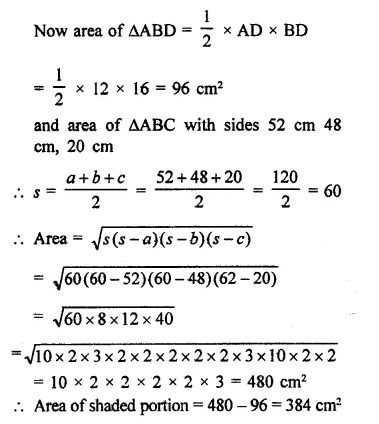Hope given RD Sharma Class 9 Solutions Chapter 17 Constructions Ex 17.1 are helpful to complete your math homework.

If you have any doubts, please comment below. Learn Insta try to provide online math tutoring for you.